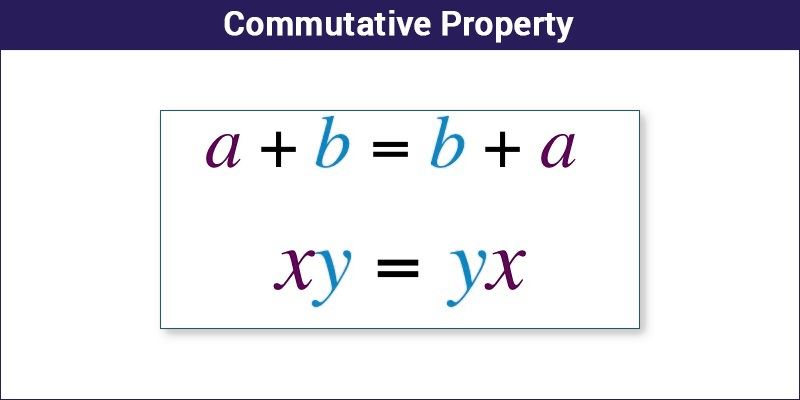# Commutative Property

In mathematical computation, commutative property or commutative law explains that order of terms doesn’t matters while performing an operation. This property is applicable only for addition and multiplication process, such that a + b = b +a and a × b = b × a. But it is not applied to subtraction and division method, because, a – b ≠ b – a and a/b ≠ b/a.

Suppose two numbers A and B on addition gives a sum C, then if we interchange the position of A and B, the result will be C only, such as; A + B = B + A = C.  For example, 4 + 3 = 7 = 3 + 4; here, whether 3 come before or after the plus sign, the sum of 4 and 3 will be always 7 irrespective of their order.

Although the official use of commutative property began at the end of the 18th century, it was known even in the ancient era. Apart from commutative, there are two more major properties of addition and multiplication of integers, they are associative and distributive. Let us discuss all the three properties or laws here.## Commutative Property Definition

There are three major properties used in the mathematical or arithmetic operations followed by integers. They are:

1. Commutative Property
2. Associative Property
3. Distributive Property

Let us discuss all the three laws or properties here.

### Commutative Law

The word, Commutative, originated from the French word ‘commute or commuter’ means to switch or move around combined with the suffix ‘-ative’ means ‘tend to’. Therefore, the literal meaning of the word is tending to switch or move around.  It states that if we swipe the positions of the integers, the result will remain the same.

According to this property, when we add two integers, the answer will remain unchanged even if the position of the numbers are changed. Let A and B are two integers, then;

Hence,

 A + B = B + A

Examples:

• 1 + 2 = 2 + 1 = 3
• 3 + 8 = 8 + 3 = 11
• 12 + 5 = 5 + 12 = 17

### Commutative Property of Multiplication

As per this property, when we multiply to two integers, the answer we get after multiplication will remain the same, even if the position of the integers are interchanged. Let A and B are two integers, then;

 A × B = B × A

Examples:

• 1 × 2 = 2 × 1 = 2
• 3 × 8 = 8 × 3 = 24
• 12 × 5 = 5 × 12 = 60

Let us see the other properties as well here:

Associative  Law of Addition and Multiplication

According to the associative law, regardless of how the numbers are grouped, you can add or multiply them. In other words, the placement of parentheses does not matter when it comes to adding or multiplying.

Hence,

 A + (B + C) = (A + B) + C A.(B.C) = (A.B).C

Distributive Law of Multiplication

The distributive property of Multiplication states that multiplying a sum by a number is the same as multiplying each addend by the value and adding the products then.

According to the Distributive Property, if a, b, c are real numbers:

a x (b + c) = (a x b) + (a x c)

## Non-Commutative Property

Some operations are non-commutative. By non-commutative, we mean the switching of the order will give different results. The mathematical operations, subtraction and division are the two non-commutative operations. Unlike addition, in subtraction switching of orders of terms results in different answers.

Example: 4 – 3 = 1 but 3 – 4 = -1 which are two different integers.

Also, division does not follow the commutative law. That is,

6 ÷ 2 = 3

2 ÷ 6 = 1/3

Hence, 6 ÷ 2 ≠  2 ÷ 6

Important Note: Commutative property works for addition and multiplication only but not for subtraction and division.

## Commutative Property Examples

Example 1: Which of the following follows commutative law?

1. 3 ×  12
2. 4 + 20
3. 36 ÷ 6
4. 36 – 6
5. -3 ×~4

Solution: Options 1, 2 and 5 follow the commutative law

Explanation:

1. 3 × 12 = 36 and

12 x 3 = 36

=> 3 x 12 = 12 x 3 (commutative)

1. 4 + 20 = 24 and

20 + 4 = 24

=> 4 + 20 = 20 + 4 (commutative)

1. 36 ÷ 6 = 6 and

6 ÷ 36 = 0.17

=> 36 ÷ 6 ≠ 6 ÷ 36  (non commutative)

1. 36 − 6 = 30 and

6 – 36 = – 30

=> 36 – 6 ≠ 6 – 36  (non commutative)

1. −3 × 4 = -12 and

4 x -3 = -12

=> −3 × 4 = 4 x -3  (commutative)

To solve more problems on properties of math, download Byju’s – The Learning App from Google Play Store and watch interactive videos.